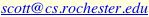# Fast Mutual Exclusion, Even With Contention

Pseudocode from UR technical report 460 (title above), by Maged M. Michael and Michael L. Scott.

These algorithms are all based on the two locks presented by Lamport in his Feb. 1987 TOCS paper. The first requires a bound on the relative rates of execution of different processes, and on the time required to execute critical sections. The second requires no such bounds, but requires space linear in the number of potentially contending processes.

Two newer fast locks require only constant space. Both require a bound on the relative rates of processes, but not on the length of critical sections. Alur and Taubenfeld's lock requires one more memory reference in the absense of contention, but Michael and Scott's lock requires the ability to read and write memory at two different (word and half-word) granularities. Both locks are appropriate for use in operating system kernels on machines that lack modern read-modify-write instructions. To satisfy the relative rates of progress assumption, they must be executed with interrupts disabled.

## Lamport's first fast lock

Code to be executed by process i. Variable `Y` is initialized to `free`. The delay at line 7 must be long enough for any process that has already read `Y = 3` at line 3 to complete lines 5, 6, and (if appropriate) 10 and 11.

```  1:  start:
2:     X := i
3:        if Y <> free
4:           goto start
5:        Y := i
6:        if X <> i
7:           { delay }
8:           if Y <> i
9:              goto start
10:    { critical section }
11:       Y := free
12:    { non-critical section }
13:       goto start
```

## Lamport's second fast lock

Code to be executed by process i. Variable `Y` is initialized to `free` and each element of the `B` array is initialized to `false`.

```  1:     start:
2:        B[i] := true
3:        X := i
4:        if Y <> free
5:           B[i] := false
6:           repeat until Y = free
7:           goto start
8:        Y := i
9:        if X <> i
10:          B[i] := false
11:          for j := 1 to N
12:             repeat while B[j]
13:          if Y <> i
14:             repeat until Y = free
15:             goto start
16:    { critical section }
17:       Y := free
18:       B[i] := false
19:    { non-critical section }
20:       goto start
```

## Alur and Taubenfeld's lock

Code to be executed by process i. Variables `Y` and `Z` are initialized to `free` and 0, respectively. The delay at line 6 is assumed to be long enough for any process that has already read `Y = free` in line 3 to complete line 4, and any process that has already set `Y` in line 4 to complete line 5 and (if not delayed) line 11 (that is the time required to service 2 memory references by all other processors in the system).

```  1:     start:
2:        X := i
3:        repeat until Y = free
4:        Y := i
5:        if X <> i
6:           { delay }
7:           if Y <> i
8:              goto start
9:           repeat until Z = 0
10:       else
11:          Z := 1
12:    { critical section }
13:       Z := 0
14:       if Y = i
15:          Y := free
16:    { non-critical section }
17:       goto start
```

## Michael and Scott's lock

Code to be executed by process i. Variables `Y` and `Z` are initialized to `free` and 0, respectively. They are assumed to occupy adjacent half-words in memory, where they can be read or written either separately or together, atomically. The delay at line 7 is assumed to be long enough for any process that has already read `Y = free` in line 3 to complete line 5, and any process that has already set `Y` in line 5 to complete line 6 and (if not delayed) line 10 (that is the time required to service 2 memory references by all other processors in the system).

```  1:     start:
2:        X := i
3:        if Y <> free
4:           goto start
5:        Y := i
6:        if X <> i
7:           { delay }
8:           if (Y,Z) <> (i,0)
9:              goto start
10:       Z := 1
11:    { critical section }
12:       (Y,Z) := (free, 0)
13:    { non-critical section }
14:       goto start
```

Last Change: 16 December 1999 /